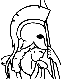#Parallel and Distributed Computation: Numerical Methods Table of Contents:

1. Introduction

1. Parallel and distributed architectures
1. The need for parallel and distributed computation
2. Parallel computing systems and their classification
2. Models, complexity measures, and some simple algorithms
1. Models
2. Complexity measures
3. Examples: Vector, and matrix computations
4. Parallelization of iterative methods
3. Communication aspects of parallel and distributed systems
3. Routing
4. Network topologies
6. Examples of matrix-vector calculations
4. Synchronization issues in parallel and distributed algorithms
1. Synchronous algorithms
2. Asynchronous algorithms and the reduction of the synchronization penalty

PART I: SYNCHRONOUS ALGORITHMS

2. Algorithms for Systems of Linear Equations and Matrix Inversion

1. Parallel algorithms for linear systems with special structure
1. Triangular matrices and back substitution
2. Tridiagonal sytems and odd-even reduction
2. Parallel direct methods for general linear equations
1. Gaussian elimination
2. Triangularization using Givens rotations
3. A fast direct matrix inversion algorithm
4. Classical iterative methods for systems of linear equations
5. Parallel implementation of classical iterative methods
1. An example: Poisson's equation
2. Multigrid methods
6. Convergence analysis of classical iterative methods
1. Background on maximum norms and nonnegative matrices
2. Convergence analysis using maximum norms
3. Convergence analysis using a quadratic cost function
4. The Poisson equation revisited
1. Description of the algorithm
2. Speed of convergence
4. Parallel implementation
8. Computation of the invariant distribution of a Markov chain
9. Fast iterative matrix inversion

3. Iterative Methods for Nonlinear Problems

1. Contraction mappings
1. General results
2. Contractions over Cartesian product sets
3. Some useful contraction mappings
2. Unconstrained optimization
1. The main algorithms
2. Convergence analysis using the descent approach
3. The case of a convex cost function
4. Nonlinear algorithms
3. Constrained convex optimization
1. Optimality conditions and the projection theorem
4. The case of a product constraint set: parallel implementations
5. Nonlinear agorithms
4. Parallelization and decomposition of optimization problems
2. Separable stritly convex programming
3. The proximal minimization algorithm
4. Augmented Lagrangian methods
5. Algorithms for variational inequalities
1. Examples of variational inequality problems
2. Preliminaries
3. The projection algorithm
4. Linearized algorithms
5. The Cartesian product case: Parallel implementations
6. Nonlinear algorithms
7. Decomposition methods for variational inequalities

4. Shortest Paths and Dynamic Programming

1. The shortest path problem
1. The Bellman-Ford algorithm
2. Other parallel shortest path methods
2. Markov chains with transition costs
3. Markovian decision problems
1. Discounted problems
2. Undiscounted problems - Stochastic shortest paths
3. Parallel implementation of the Dynamic Programming iteration

5. Network Flow Problems

1. The linear network flow problem and its dual
2. The relaxation method
1. Application to the shortest path problem
2. Multiple node relaxation method
3. The epsilon-relaxation method
1. The auction algorithm for the assignment problem
2. Parallel versions of the epsilon-relaxation and the auction algorithms
4. Complexity analysis of the epsilon-relaxation method and its scaled version
1. The scaled version of the algorithm
2. Application to the assignment problem
5. Network flow problems with strictly convex cost
1. The relaxation method
2. Convergence analysis
3. The problem without arc flow bounds
4. An example: Constrained matrix problems
5. Parallel implementations of the relaxation method
6. Nonlinear multicommodity flow problems - Routing applications

PART II: ASYNCHRONOUS ALGORITHMS

6. Totally Asynchronous Iterative Methods

1. The totally asynchronous algorithmic model
2. A general convergence theorem
3. Applications to problems involving maximum norm contraction mappings
1. Solution of linear systems of equations
2. Unconstrained optimization
3. Constrained optimization and variational inequalities
4. Dynamic programming
5. Convergence rate comparison of synchronous and asynchronous algorithms
4. Applications to monotone mappings and the shortest path problem
5. Linear network flow problems
6. Nonlinear network flow problems
7. Asynchronous relaxation for ordinary differential equations and two--point boundary value problems
1. The asynchronous relaxation algorithm
2. Two-point boundary value problems
3. The discrete time case

7. Partially Asynchronous Iterative Methods

1. The partially asynchronous algorithmic model
2. Algorithms for fixed points of nonexpansive mappings
1. A convergence result
2. Weakly diagonally dominant systems of linear equations
3. Strictly convex network flow problems
3. Algorithms for agreement and for Markov chain problems
1. The agreement algorithm
2. An asynchronous algorithm for the invariant distribution of a Markov chain
4. Load balancing in a computer network
1. The algorithm and its convergence
2. The role of the various parameters
3. Block - iterative algorithms
6. Distributed asynchronous routing in data networks
1. Problem definition
2. The algorihm and its convergence
3. Discussion
7. A model in which several processors may update the same variables
1. Description of the algorithm and assumptions
2. A convergence result
3. Discussion and extensions

8. Organizing an Asynchronous Network of Processors for Distributed Computation

1. Detecting termination of a distributed algorithm
2. Snapshots
3. Resource scheduling
4. Synchronization using rollback: asynchronous simulation
5. Maintaining communication with a center

9. APPENDIXES

1. Appendix A: Linear algebra and analysis
2. Appendix B: Graph theory
3. Appendix C: Optimization and duality theory
4. Appendix D: Probability theory and Markov chains